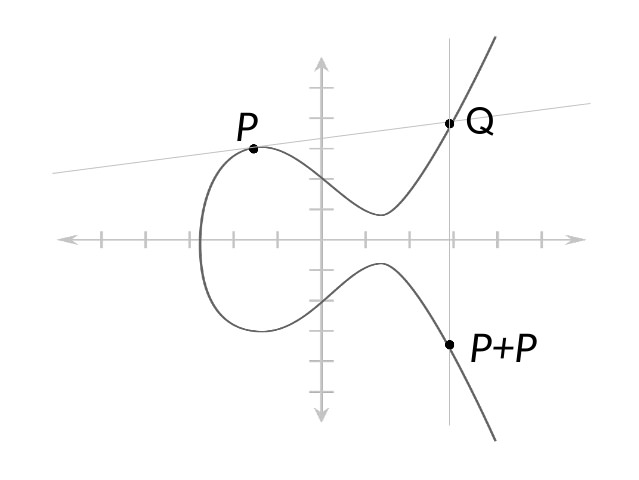# Halloween Party - ASIS CTF Quals 2019

tl;dr

1. Find Elliptic Curve parameters from given points on the curve
2. Find x-coordinate of 2*P, given y-coordinate of 2*P
3. Invert 2 over mod (P.order()) and multiply the result with 2*P to get P
4. Submit ASIS{P.x} as the flag

Challenge Points: 182
Challenge Solves: 20
Solved by: s0rc3r3r, v3ct0r, v4d3r

In case you are new to Elliptic Curves, you can read about them in my library here

## Preliminary Analysis

We are given a script that implements a basic Elliptic Curve (Weierstraas model)
$y^2 \equiv x^3 + a*x + b\mod p$:

The script is importing EC parameters from a secret file, hence our first task becomes recovering the
Elliptic Curve parameters.

Notice that we are given three points on the curve: P1, P2 and P3, coordinates of which are known to us.
We can use these points to get the values of curve parameters.

## Retrieving EC parameters

Let $P1 = (x_1, y_1)$, $P2 = (x_2, y_2)$ & $P3 = (x_3, y_3)$
This implies:
$y_1^2 = x_1^3 + a*x_1 + b \mod p$
$y_2^2 = x_2^3 + a*x_2 + b \mod p$
$y_3^2 = x_3^3 + a*x_3 + b \mod p$

Substituting values of P1 = (x1, y1), P2 = (x2, y2) & P3 = (x3, y3) and solving for a, b, p, we get:

There are a few assertions in the challenge script:

As one can see, our goal is to find the x-coordinate of P, which is the flag.

It is given that x-coordinate of P’ = 2*P (scalar multiplication) is equal to x-coordinate of Q. Note, this can
only happen when y-coordinate of Q is an additive inverse of y-coordinate of P’.

Let us try to understand the reason behind the above:

1. Consider an Elliptic Curve $y^2\equiv x^3 + a*x + b\mod p$,
2. If two points A = (x1, y1) and B = (x2, y2) have same x-coordinate, then we can write:
• $y_1^2 \equiv c\mod p$
• $y_2^2 \equiv c\mod p$
3. Provided y1 != y2, we can say that $y_1 \equiv -y_2 \mod p$ as:
• $y_1^2 \equiv (-y_2)^2 \equiv c\mod p$

We are given y-coordinate of (-1)*Q, which implies that we are given the y-coordinate of P’

On the basis of observations above, we can draw the Elliptic Curve and mark relevant points:Note that the above diagram is made for Curve over $\mathbb{R}$, whereas our Curve is defined over $GF(p)$,
but the idea remains the same! Also, $P+P == 2*P$

## Finding x-coordinate of Q

Provided y-coordinate of a point on the curve, we can compute it’s corresponding x-coordinate by finding
solutions of the equation: $x^3 + a*x + (b-y^2) \equiv 0 \mod p$

Values of a, b, p in our challenge are as follows:

We computed the x-coordinate of Q by running the following function in Mathematica:

Alternatively, you can also use roots() in sagemath:

Source: HATS SG team’s solution script - https://pastebin.com/cUAa6R9W

Recovered x-coordinate of Q as: 708927573459527177103235542148826237228344428002
Since x-coordinate of Q and that of P’=2*P is the same, we have both x and y-coordinates of P’.

Knowledge of both coordinates of 2*P would be adequate to get coordinates of P

## Finding x-coordinate of P

Let the order of subgroup generated by P be ordP. We can then write:
$2*P = P’$
$P = ({2^{-1}\mod ord_P})*P’$

But, how do we find order of subgroup generated by P?

We know from Lagrange’s Theorem that order of the subgroup generated by a point P on the curve is a factor of cardinality of the curve.

But if P is a generator, then order of the subgroup generated by P is exactly equal to the cardinality of
the curve. We can find out cardinality of a curve E using the following function in sagemath:

Cardinality of our curve has several factors: [3, 5, 13, 257, 134021890447, 97090721460179,
1354215209508238123] and order of P can be a factor of combination of any of these factors.

But we went on further anyway, assuming that P is a generator for the curve given in this challenge and got
the correct coordinates :)

You can read the full solution script here:

On running the above code, we got the coordinates of P as: (804028439497151963978256498500182891314861988389, 272052071247970914287181631972199909106801861256)

Flag: ASIS{804028439497151963978256498500182891314861988389}

In case of any query, feedback or suggestion, feel free to comment or reach me out on Twitter!